# SVM Simulation for three level inverter

Jun. 28, 2014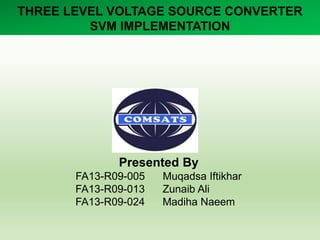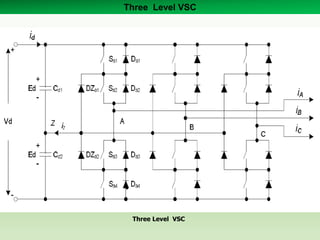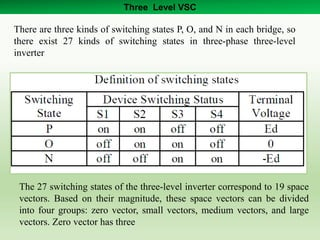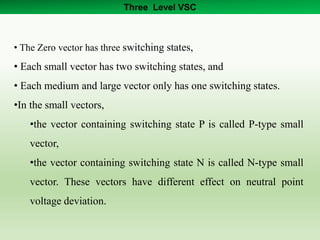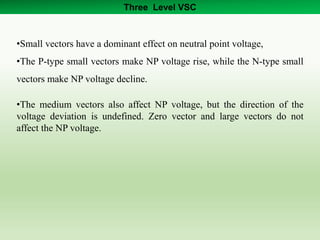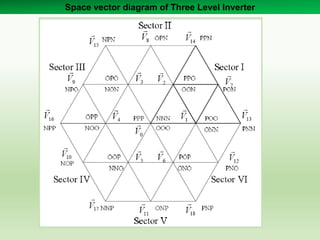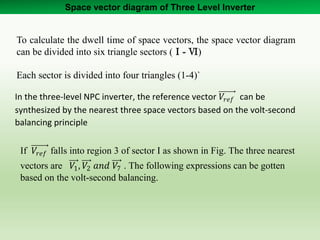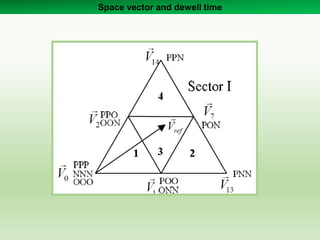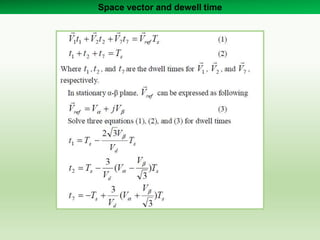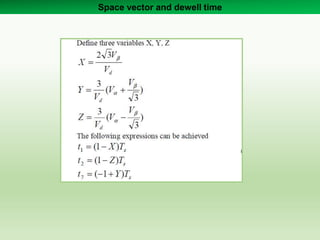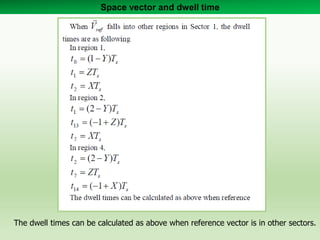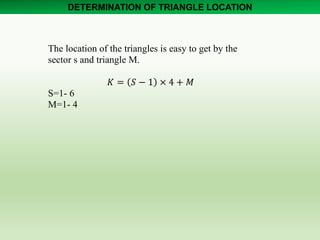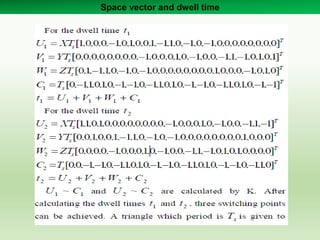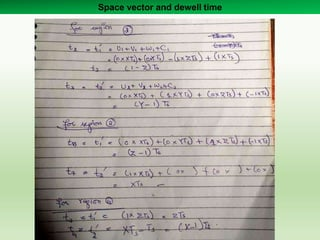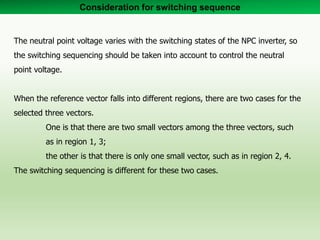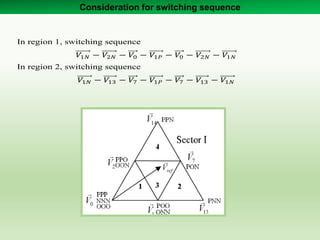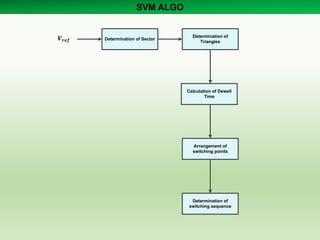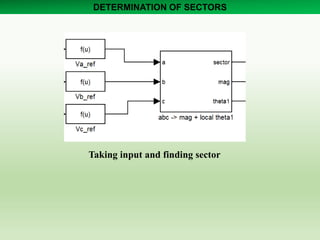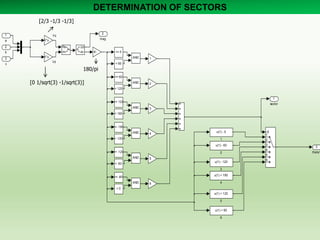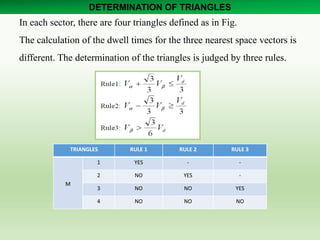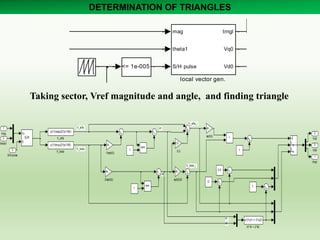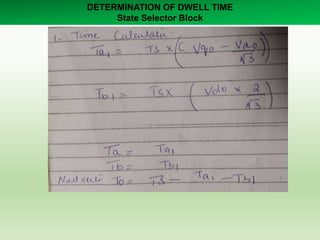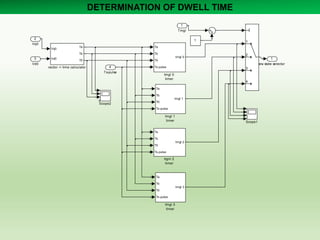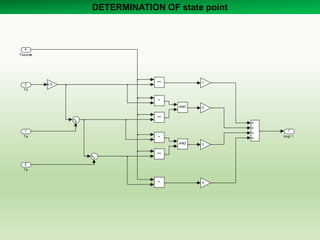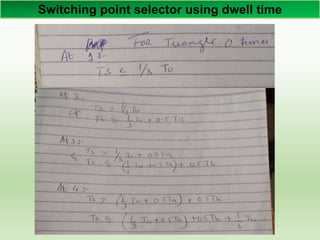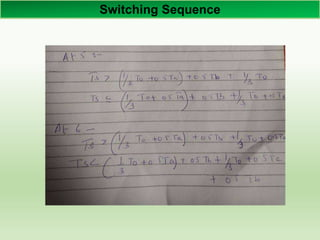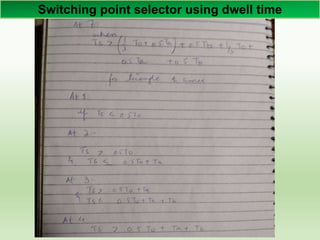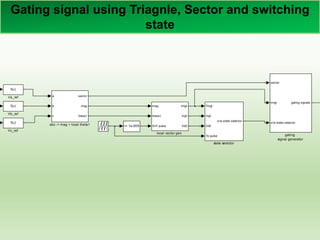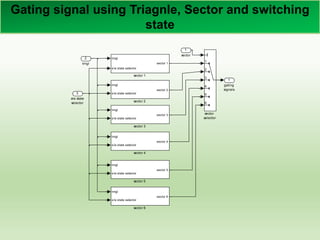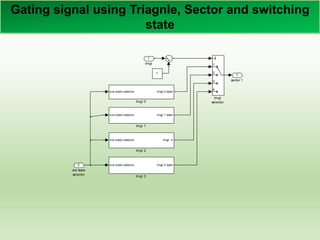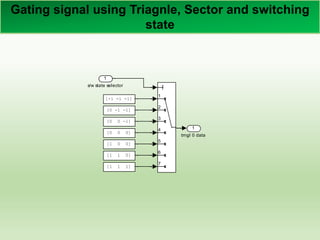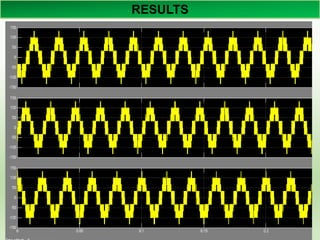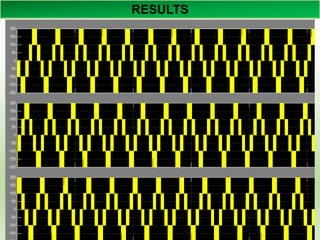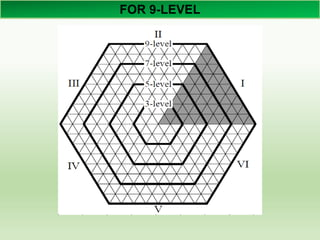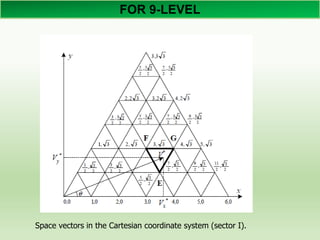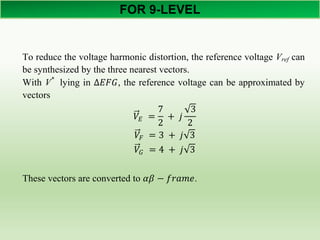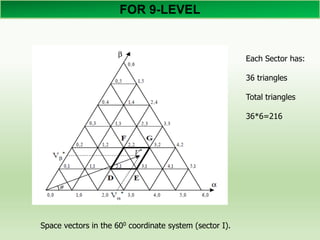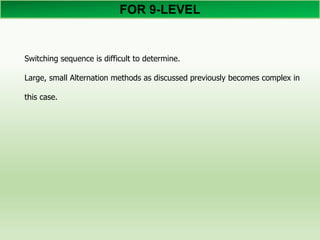1 of 38

### SVM Simulation for three level inverter

1. THREE LEVEL VOLTAGE SOURCE CONVERTER SVM IMPLEMENTATION Presented By FA13-R09-005 Muqadsa Iftikhar FA13-R09-013 Zunaib Ali FA13-R09-024 Madiha Naeem
2. Three Level VSC Three Level VSC
3. Three Level VSC The 27 switching states of the three-level inverter correspond to 19 space vectors. Based on their magnitude, these space vectors can be divided into four groups: zero vector, small vectors, medium vectors, and large vectors. Zero vector has three There are three kinds of switching states P, O, and N in each bridge, so there exist 27 kinds of switching states in three-phase three-level inverter
4. Three Level VSC • The Zero vector has three switching states, • Each small vector has two switching states, and • Each medium and large vector only has one switching states. •In the small vectors, •the vector containing switching state P is called P-type small vector, •the vector containing switching state N is called N-type small vector. These vectors have different effect on neutral point voltage deviation.
5. Three Level VSC •Small vectors have a dominant effect on neutral point voltage, •The P-type small vectors make NP voltage rise, while the N-type small vectors make NP voltage decline. •The medium vectors also affect NP voltage, but the direction of the voltage deviation is undefined. Zero vector and large vectors do not affect the NP voltage.
6. Space vector diagram of Three Level Inverter
7. Space vector diagram of Three Level Inverter To calculate the dwell time of space vectors, the space vector diagram can be divided into six triangle sectors (Ⅰ- Ⅵ) Each sector is divided into four triangles (1-4)` In the three-level NPC inverter, the reference vector 𝑉𝑟𝑒𝑓 can be synthesized by the nearest three space vectors based on the volt-second balancing principle If 𝑉𝑟𝑒𝑓 falls into region 3 of sector I as shown in Fig. The three nearest vectors are 𝑉1, 𝑉2 𝑎𝑛𝑑 𝑉7 . The following expressions can be gotten based on the volt-second balancing.
8. Space vector and dewell time
9. Space vector and dewell time
10. Space vector and dewell time
11. Space vector and dwell time The dwell times can be calculated as above when reference vector is in other sectors.
12. DETERMINATION OF TRIANGLE LOCATION The location of the triangles is easy to get by the sector s and triangle M. 𝐾 = 𝑆 − 1 × 4 + 𝑀 S=1- 6 M=1- 4
13. Space vector and dwell time
14. Space vector and dewell time
15. Consideration for switching sequence The neutral point voltage varies with the switching states of the NPC inverter, so the switching sequencing should be taken into account to control the neutral point voltage. When the reference vector falls into different regions, there are two cases for the selected three vectors. One is that there are two small vectors among the three vectors, such as in region 1, 3; the other is that there is only one small vector, such as in region 2, 4. The switching sequencing is different for these two cases.
16. Consideration for switching sequence In region 1, switching sequence 𝑉1𝑁 − 𝑉2𝑁 − 𝑉0 − 𝑉1𝑃 − 𝑉0 − 𝑉2𝑁 − 𝑉1𝑁 In region 2, switching sequence 𝑉1𝑁 − 𝑉13 − 𝑉7 − 𝑉1𝑃 − 𝑉7 − 𝑉13 − 𝑉1𝑁
17. SVM ALGO Determination of Sector Determination of Triangles Calculation of Dewell Time Arrangement of switching points Determination of switching sequence
18. DETERMINATION OF SECTORS Taking input and finding sector
19. DETERMINATION OF SECTORS Vq Vd 3 theta1 2 mag 1 sector Re Im 1 2 3 4 5 6 AND AND AND AND AND AND 6 5 4 3 2 1 -K- K*u K*u |u| u < -60 >= -120 < -120 >= -180 < 180 >= 120 < 120 >= 60 < 0 >= -60 < 60 >= 0 u(1) + 60 6 u(1) + 120 5 u(1) + 180 4 u(1) - 120 3 u(1) - 60 2 u(1) - 0 1 3 c 2 b 1 a [2/3 -1/3 -1/3] [0 1/sqrt(3) -1/sqrt(3)] 180/pi
20. DETERMINATION OF TRIANGLES In each sector, there are four triangles defined as in Fig. The calculation of the dwell times for the three nearest space vectors is different. The determination of the triangles is judged by three rules. TRIANGLES RULE 1 RULE 2 RULE 3 M 1 YES - - 2 NO YES - 3 NO NO YES 4 NO NO NO
21. DETERMINATION OF TRIANGLES k2 k1 V_beta_i V_alfa_i V_beta V_alfa k2 k1 3 Vd0 2 Vq0 1 trngl -K- sqrt(3)/2 -K- sqrt(3) 1 0.5 -C- 1 1 1 u(1)*u(1) + 2*u(2) k1*k1 + 2*k2 u(1)*sin(u(2)*pi/180) V_beta u(1)*cos(u(2)*pi/180) V_alfa In S S/H > 1 2 rem rem -K- 2/sqrt(3) -K- 1/sqrt(3) 0.5 0.53 S/H pulse 2 theta1 1 mag Trngl Vq0 Vd0 Ts-pulse s/w state sele state selector mag theta1 S/H pulse trngl Vq0 Vd0 local vector gen. gm CE ga9 g C gm CE ga5 g C a b c sector mag theta1 c -> mag + local theta1 [gc2] [gc1] [gb2] m k Db3 <= 1e-005 Taking sector, Vref magnitude and angle, and finding triangle
22. DETERMINATION OF DWELL TIME State Selector Block
23. DETERMINATION OF DWELL TIME 1 s/w state selector Vq0 Vd0 Ta Tb T0 vector -> time calculator Ta Tb T0 Ts-pulse trngl 3 trngl 3 timer Ta Tb T0 Ts-pulse trngl 1 trngl 1 timer Ta Tb T0 Ts-pulse trngl 0 trngl 0 timer Ta Tb T0 Ts-pulse trngl 2 trgnl 2 timer 1 Scope2 Scope1 1 2 3 4 4 Ts-pulse 3 Vd0 2 Vq0 1 Trngl
24. DETERMINATION OF state point 1 trngl 1 > <= > <= > <= AND AND 4 3 2 10.5 4 Ts-pulse 3 T0 2 Tb 1 Ta
25. Switching point selector using dwell time
26. Switching Sequence
27. Switching point selector using dwell time
28. Gating signal using Triagnle, Sector and switching state Trngl Vq0 Vd0 Ts-pulse s/w state selector state selector mag theta1 S/H pulse trngl Vq0 Vd0 local vector gen. sector trngl s/w state selector gating signals gating signal generator gm CE ga9 gm CE ga6 gm CE ga5 gm CE 2 gm CE ga10 gm CE 1 a b c sector mag theta1 abc -> mag + local theta1 f(u) Vc_ref f(u) Vb_ref f(u) Va_ref Scope1 [gc2] [gc1] [gb2] [gb1] m a k Db3 m a k Db1 <= 1e-005
29. Gating signal using Triagnle, Sector and switching state 1 gating signals trngl s/w state selector sector 6 sector 6 trngl s/w state selector sector 5 sector 5 trngl s/w state selector sector 4 sector 4 trngl s/w state selector sector 3 sector 3 trngl s/w state selector sector 2 sector 2 trngl s/w state selector sector 1 sector 1 1 2 3 4 5 6 sector selector 3 s/w state selector 2 trngl 1 sector
30. Gating signal using Triagnle, Sector and switching state 1 sector 1 s/w state selector trngl 3 data trngl 3 s/w state selector trngl 2 trngl 2 s/w state selector trngl 1 data trngl 1 s/w state selector trngl 0 data trngl 0 1 2 3 4 trngl selector 1 2 s/w state selector 1 trngl
31. Gating signal using Triagnle, Sector and switching state 1 trngl 0 data [1 0 0] [-1 -1 -1] [1 1 1] [1 1 0] [0 0 0] [0 0 -1] [0 -1 -1] 1 2 3 4 5 6 7 1 s/w state selector
32. RESULTS
33. RESULTS
34. FOR 9-LEVEL
35. FOR 9-LEVEL Space vectors in the Cartesian coordinate system (sector I).
36. FOR 9-LEVEL To reduce the voltage harmonic distortion, the reference voltage Vref can be synthesized by the three nearest vectors. With V* lying in ∆𝐸𝐹𝐺, the reference voltage can be approximated by vectors 𝑉𝐸 = 7 2 + 𝑗 3 2 𝑉𝐹 = 3 + 𝑗 3 𝑉𝐺 = 4 + 𝑗 3 These vectors are converted to 𝛼𝛽 − 𝑓𝑟𝑎𝑚𝑒.
37. FOR 9-LEVEL Space vectors in the 600 coordinate system (sector I). Each Sector has: 36 triangles Total triangles 36*6=216
38. FOR 9-LEVEL Switching sequence is difficult to determine. Large, small Alternation methods as discussed previously becomes complex in this case.